GeeksforGeeks App
Open AppBrowser
Continue

# How to add a new column to a PySpark DataFrame ?

Create the first data frame for demonstration:

Here, we will be creating the sample data frame which we will be used further to demonstrate the approach purpose.

## Python3

 `# importing module``import` `pyspark` `# importing sparksession from pyspark.sql module``from` `pyspark.sql ``import` `SparkSession` `# creating sparksession and giving an app name``spark ``=` `SparkSession.builder.appName(``'sparkdf'``).getOrCreate()` `# list  of employee data``data ``=` `[[``"1"``, ``"sravan"``, ``"company 1"``],``        ``[``"2"``, ``"ojaswi"``, ``"company 1"``],``        ``[``"3"``, ``"rohith"``, ``"company 2"``],``        ``[``"4"``, ``"sridevi"``, ``"company 1"``],``        ``[``"5"``, ``"bobby"``, ``"company 1"``]]` `# specify column names``columns ``=` `[``'ID'``, ``'NAME'``, ``'Company'``]` `# creating a dataframe from the lists of data``dataframe ``=` `spark.createDataFrame(data, columns)` `dataframe.show()`

Output: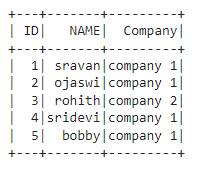## Method 1: Add New Column With Constant Value

In this approach to add a new column with constant values, the user needs to call the lit() function parameter of the withColumn() function and pass the required parameters into these functions. Here, the lit() is available in pyspark.sql. Functions module.

Syntax:

`dataframe.withColumn("column_name", lit(value))`

where,

• dataframe is the pyspark input dataframe
• column_name is the new column to be added
• value is the constant value to be assigned to this column

Example:

In this example, we add a column named salary with a value of 34000 to the above dataframe using the withColumn() function with the lit() function as its parameter in the python programming language.

## Python3

 `# importing module``import` `pyspark` `# import lit function``from` `pyspark.sql.functions ``import` `lit` `# importing sparksession from pyspark.sql module``from` `pyspark.sql ``import` `SparkSession` `# creating sparksession and giving an app name``spark ``=` `SparkSession.builder.appName(``'sparkdf'``).getOrCreate()` `# list  of employee data``data ``=` `[[``"1"``, ``"sravan"``, ``"company 1"``],``        ``[``"2"``, ``"ojaswi"``, ``"company 1"``],``        ``[``"3"``, ``"rohith"``, ``"company 2"``],``        ``[``"4"``, ``"sridevi"``, ``"company 1"``],``        ``[``"5"``, ``"bobby"``, ``"company 1"``]]` `# specify column names``columns ``=` `[``'ID'``, ``'NAME'``, ``'Company'``]` `# creating a dataframe from the lists of data``dataframe ``=` `spark.createDataFrame(data, columns)` `# Add a column named salary with value as 34000``dataframe.withColumn(``"salary"``, lit(``34000``)).show()`

Output: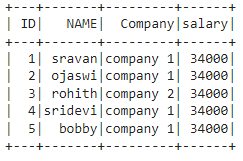## Method 2: Add Column Based on Another Column of DataFrame

Under this approach, the user can add a new column based on an existing column in the given dataframe.

### Example 1: Using withColumn() method

Here, under this example, the user needs to specify the existing column using the withColumn() function with the required parameters passed in the python programming language.

Syntax:

`dataframe.withColumn("column_name", dataframe.existing_column)`

where,

• dataframe is the input dataframe
• column_name is the new column
• existing_column is the column which is existed

In this example, we are adding a column named salary from the ID column with multiply of 2300 using the withColumn() method in the python language,

## Python3

 `# importing module``import` `pyspark` `# import lit function``from` `pyspark.sql.functions ``import` `lit` `# importing sparksession from pyspark.sql module``from` `pyspark.sql ``import` `SparkSession` `# creating sparksession and giving an app name``spark ``=` `SparkSession.builder.appName(``'sparkdf'``).getOrCreate()` `# list  of employee data``data ``=` `[[``"1"``, ``"sravan"``, ``"company 1"``],``        ``[``"2"``, ``"ojaswi"``, ``"company 1"``],``        ``[``"3"``, ``"rohith"``, ``"company 2"``],``        ``[``"4"``, ``"sridevi"``, ``"company 1"``],``        ``[``"5"``, ``"bobby"``, ``"company 1"``]]` `# specify column names``columns ``=` `[``'ID'``, ``'NAME'``, ``'Company'``]` `# creating a dataframe from the lists of data``dataframe ``=` `spark.createDataFrame(data, columns)` `# Add a column named salary from ID column with multiply of 2300``dataframe.withColumn(``"salary"``, dataframe.``ID``*``2300``).show()`

Output: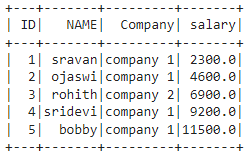### Example 2 : Using concat_ws()

Under this example, the user has to concat the two existing columns and make them as a new column by importing this method from pyspark.sql.functions module.

Syntax:

dataframe.withColumn(“column_name”, concat_ws(“Separator”,”existing_column1″,’existing_column2′))

where,

• dataframe is the input dataframe
• column_name is the new column name
• existing_column1 and existing_column2 are the two columns to be added with Separator to make values to the new column
• Separator is like the operator between values with two columns

Example:

In this example, we add a column named Details from Name and Company columns separated by “-” in the python language.

## Python3

 `# importing module``import` `pyspark` `# import concat_ws function``from` `pyspark.sql.functions ``import` `concat_ws` `# importing sparksession from pyspark.sql module``from` `pyspark.sql ``import` `SparkSession` `# creating sparksession and giving an app name``spark ``=` `SparkSession.builder.appName(``'sparkdf'``).getOrCreate()` `# list  of employee data``data ``=` `[[``"1"``, ``"sravan"``, ``"company 1"``],``        ``[``"2"``, ``"ojaswi"``, ``"company 1"``],``        ``[``"3"``, ``"rohith"``, ``"company 2"``],``        ``[``"4"``, ``"sridevi"``, ``"company 1"``],``        ``[``"5"``, ``"bobby"``, ``"company 1"``]]` `# specify column names``columns ``=` `[``'ID'``, ``'NAME'``, ``'Company'``]` `# creating a dataframe from the lists of data``dataframe ``=` `spark.createDataFrame(data, columns)` `# Add a column named Details from Name and Company columns separated by -``dataframe.withColumn(``"Details"``, concat_ws(``"-"``, ``"NAME"``, ``'Company'``)).show()`

Output: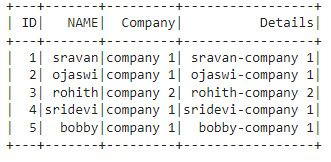## Method 3: Add Column When not Exists on DataFrame

In this method, the user can add a column when it is not existed by adding a column with the lit() function and checking using if the condition.

Syntax:

```if 'column_name' not in dataframe.columns:
dataframe.withColumn("column_name",lit(value))```

where,

• dataframe. columns are used to get the column names

Example:

In this example, we add a column of the salary to 34000 using the if condition with the withColumn() and the lit() function.

## Python3

 `# importing module``import` `pyspark` `# import concat_ws and lit function``from` `pyspark.sql.functions ``import` `concat_ws, lit` `# importing sparksession from pyspark.sql module``from` `pyspark.sql ``import` `SparkSession` `# creating sparksession and giving an app name``spark ``=` `SparkSession.builder.appName(``'sparkdf'``).getOrCreate()` `# list  of employee data``data ``=` `[[``"1"``, ``"sravan"``, ``"company 1"``],``        ``[``"2"``, ``"ojaswi"``, ``"company 1"``],``        ``[``"3"``, ``"rohith"``, ``"company 2"``],``        ``[``"4"``, ``"sridevi"``, ``"company 1"``],``        ``[``"5"``, ``"bobby"``, ``"company 1"``]]` `# specify column names``columns ``=` `[``'ID'``, ``'NAME'``, ``'Company'``]` `# creating a dataframe from the lists of data``dataframe ``=` `spark.createDataFrame(data, columns)` `# add salary column by checking its existence``if` `'salary'` `not` `in` `dataframe.columns:``    ``dataframe.withColumn(``"salary"``, lit(``34000``)).show()`

Output: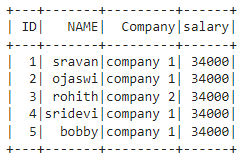## Method 4: Add Column to DataFrame using select()

In this method, to add a column to a data frame, the user needs to call the select() function to add a column with lit() function and select() method. It will also display the selected columns.

Syntax:

`dataframe.select(lit(value).alias("column_name"))`

where,

• dataframe is the input dataframe
• column_name is the new column

Example:

In this example, we add a salary column with a constant value of 34000 using the select() function with the lit() function as its parameter.

## Python3

 `# importing module``import` `pyspark` `# import concat_ws and lit function``from` `pyspark.sql.functions ``import` `concat_ws, lit` `# importing sparksession from pyspark.sql module``from` `pyspark.sql ``import` `SparkSession` `# creating sparksession and giving an app name``spark ``=` `SparkSession.builder.appName(``'sparkdf'``).getOrCreate()` `# list  of employee data``data ``=` `[[``"1"``, ``"sravan"``, ``"company 1"``],``        ``[``"2"``, ``"ojaswi"``, ``"company 1"``],``        ``[``"3"``, ``"rohith"``, ``"company 2"``],``        ``[``"4"``, ``"sridevi"``, ``"company 1"``],``        ``[``"5"``, ``"bobby"``, ``"company 1"``]]` `# specify column names``columns ``=` `[``'ID'``, ``'NAME'``, ``'Company'``]` `# creating a dataframe from the lists of data``dataframe ``=` `spark.createDataFrame(data, columns)` `# add salary column with constant value - 34000``dataframe.select(lit(``34000``).alias(``"salary"``)).show()`

Output: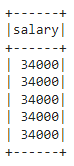## Method 5: Add Column to DataFrame using SQL Expression

In this method, the user has to use SQL expression with SQL function to add a column. Before that, we have to create a temporary view, From that view, we have to add and select columns.

Syntax:

```dataframe.createOrReplaceTempView("name")
spark.sql("select 'value' as column_name from view")```

where,

• dataframe is the input dataframe
• name is the temporary view name
• sql function will take SQL expression as input to add a column
• column_name is the new column name
• value is the column value

Example:

Add new column named salary with 34000 value

## Python3

 `# importing module``import` `pyspark` `# import concat_ws and lit function``from` `pyspark.sql.functions ``import` `concat_ws, lit` `# importing sparksession from pyspark.sql module``from` `pyspark.sql ``import` `SparkSession` `# creating sparksession and giving an app name``spark ``=` `SparkSession.builder.appName(``'sparkdf'``).getOrCreate()` `# list  of employee data``data ``=` `[[``"1"``, ``"sravan"``, ``"company 1"``],``        ``[``"2"``, ``"ojaswi"``, ``"company 1"``],``        ``[``"3"``, ``"rohith"``, ``"company 2"``],``        ``[``"4"``, ``"sridevi"``, ``"company 1"``],``        ``[``"5"``, ``"bobby"``, ``"company 1"``]]` `# specify column names``columns ``=` `[``'ID'``, ``'NAME'``, ``'Company'``]` `# creating a dataframe from the lists of data``dataframe ``=` `spark.createDataFrame(data, columns)` `# create view``dataframe.createOrReplaceTempView(``"view"``)` `# add new column named salary with 34000 value``spark.sql(``"select '34000' as salary from view"``).show()`

Output:## Method 6: Add Column Value Based on Condition

Under this method, the user needs to use the when function along with withcolumn() method used to check the condition and add the column values based on existing column values. So we have to import when() from pyspark.sql.functions to add a specific column based on the given condition.

Syntax:

dataframe.withColumn(“column_name”,

when((dataframe.column_name condition1), lit(“value1”)).

when((dataframe.column_name condition2), lit(“value2”)).

———————

———————

when((dataframe.column_name conditionn), lit(“value3”)).

.otherwise(lit(“value”)) )

where,

• column_name is the new column name
• condition1 is the condition to check and assign value1 using lit() through when
• otherwise, it is the keyword used to check when no condition satisfies.

Example:

In this example, we add a new column named salary and add value 34000 when the name is sravan and add value 31000 when the name is ojsawi, or bobby otherwise adds 78000 using the when() and the withColumn() function.

## Python3

 `# importing module``import` `pyspark` `# import when and lit function``from` `pyspark.sql.functions ``import` `when, lit` `# importing sparksession from pyspark.sql module``from` `pyspark.sql ``import` `SparkSession` `# creating sparksession and giving an app name``spark ``=` `SparkSession.builder.appName(``'sparkdf'``).getOrCreate()` `# list  of employee data``data ``=` `[[``"1"``, ``"sravan"``, ``"company 1"``],``        ``[``"2"``, ``"ojaswi"``, ``"company 1"``],``        ``[``"3"``, ``"rohith"``, ``"company 2"``],``        ``[``"4"``, ``"sridevi"``, ``"company 1"``],``        ``[``"5"``, ``"bobby"``, ``"company 1"``]]` `# specify column names``columns ``=` `[``'ID'``, ``'NAME'``, ``'Company'``]` `# creating a dataframe from the lists of data``dataframe ``=` `spark.createDataFrame(data, columns)` `# add a new column named salary``# add value 34000 when name is sravan``# add value 31000 when name is ojsawi or bobby``# otherwise add 78000``dataframe.withColumn(``"salary"``,``                     ``when((dataframe.NAME ``=``=` `"sravan"``), lit(``"34000"``)).``                     ``when((dataframe.NAME ``=``=` `"ojsawi"``) | (``                         ``dataframe.NAME ``=``=` `"bobby"``), lit(``"31000"``))``                     ``.otherwise(lit(``"78000"``))).show()`

Output: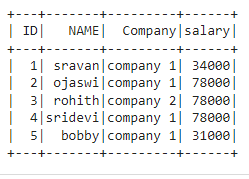My Personal Notes arrow_drop_up